# Geometry Contest!

Rules of the contest:

1.Suppose problem $i$ is posted. The one who solves the problem and posts the solution becomes able to publish the $i+1$ problem.

2.This will continue until the problem posted is not answered within $2$ hours. If the solution is not posted, the problem maker himself starts with the next problem, posting a solution of the previous one.

3.The deadline question will be declared later. Meaning, the question with which the contest ends.

4.Whosoever posts a new problem should post on slack in #general that new problem is up!

5.The new problem poster should know the solution of his posted problem.

6.If the new problem is not posted in 15 minutes of answering the previous question, ANYBODY can post a new question.

7.Marking scheme is none, This time the question setter will decide the marks, Out of 5 and the one who answers will be given that credit. The marks will be set acc. To the level of the question as in brilliant!

8.We are starting from Question 1.

Points table:

1.Prince Loomba:8 marks

2.Julian Poon:5 marks

3..Michael Fuller:4 marks

4.Ayush Rai:3 marks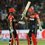Note by Ayush G Rai
4 years, 9 months ago

This discussion board is a place to discuss our Daily Challenges and the math and science related to those challenges. Explanations are more than just a solution — they should explain the steps and thinking strategies that you used to obtain the solution. Comments should further the discussion of math and science.

When posting on Brilliant:

• Use the emojis to react to an explanation, whether you're congratulating a job well done , or just really confused .
• Ask specific questions about the challenge or the steps in somebody's explanation. Well-posed questions can add a lot to the discussion, but posting "I don't understand!" doesn't help anyone.
• Try to contribute something new to the discussion, whether it is an extension, generalization or other idea related to the challenge.

MarkdownAppears as
*italics* or _italics_ italics
**bold** or __bold__ bold
- bulleted- list
• bulleted
• list
1. numbered2. list
1. numbered
2. list
Note: you must add a full line of space before and after lists for them to show up correctly
paragraph 1paragraph 2

paragraph 1

paragraph 2

[example link](https://brilliant.org)example link
> This is a quote
This is a quote
    # I indented these lines
# 4 spaces, and now they show
# up as a code block.

print "hello world"
# I indented these lines
# 4 spaces, and now they show
# up as a code block.

print "hello world"
MathAppears as
Remember to wrap math in $$ ... $$ or $ ... $ to ensure proper formatting.
2 \times 3 $2 \times 3$
2^{34} $2^{34}$
a_{i-1} $a_{i-1}$
\frac{2}{3} $\frac{2}{3}$
\sqrt{2} $\sqrt{2}$
\sum_{i=1}^3 $\sum_{i=1}^3$
\sin \theta $\sin \theta$
\boxed{123} $\boxed{123}$

Sort by:

- 4 years, 9 months ago

i want to take part in this where is the present question

- 4 years, 9 months ago

goto the comments sorting option and click New, instead of Top. Anyways, Problem 9 is most recent. No one has solved properly.

- 4 years, 9 months ago

Problem 9 [5 marks]

Let $X$ and $Y$ be points inside equilateral triangle $ABC$. Let $Y'$ be the reflection of $Y$ in line $BC$. Prove that

$XY+XB+XC \geq Y'A$

- 4 years, 9 months ago

I have given you 10 cases

- 4 years, 9 months ago

Ok I will try more cases.

See this,

IF X=Y=B or X=C=Y then the equation holds true that LHS=RHS.

IF X=Y is close to B or C the equation holds true that LHS>RHS

IF X=Y=A then the equation holds that LHS>RHS

IF X=Y is close to A then the equation holds that LHS>RHS

IF X=B or is close to B and Y=/=X and is far from B then the equation holds that LHS>RHS

IF X=B or is close to B and Y=/=X and is near B then the equation holds that LHS>RHS

IF X=C or is close to C and Y=/=X and is far from B then the equation holds that LHS>RHS

IF X=C or is close to C and Y=/=X and is near B then the equation holds that LHS>RHS.

IF X=centroid or is close to B and Y=/=X and is far from the centroid then the equation holds that LHS>RHS

IF X=centroid or is close to B and Y=/=X and is near the centroid then the equation holds that LHS>RHS

So LHS is greater than or equal to RHS

Done.

- 4 years, 9 months ago

You will have to prove 'in geenral ' , not case by case coz there are infinite cases.

- 4 years, 9 months ago

all types of cases are part of these cases though.

- 4 years, 9 months ago

https://i.imgur.com/xmrurud.png

- 4 years, 9 months ago

come to https://brilliant.org/discussions/thread/brilliant-geometry-contest-season-1/.This problem is shifted there.

- 4 years, 9 months ago

Ok I will still try to prove AGAIN using scenarios.

Ok so let's first minimise XY+XB+XC. Suppose X=B=Y then XB=XY=0 OR X=C=Y then XC=XY=0. We know that AB=AC=BC=B'C. Then X'=Y'=B' or X'=Y'=C. So A is the furthest point from X and Y. I will prove that the the worst scenario of LHS is the best scenario of RHS. Ok I will let X be a tpont B (works for C too). Since X=B=Y, XB=XY=0 and XY+XB+XC=XC=BC only. Now the furthest length from A that Y can be at is at point X which is either Point B or C. YA=Y'A=BC=AB=AC=XY+XB=XC. So it is possible that XY+XB+XC=Y'A. -eq(1)

Now to prove that LHS can be greater than RHS, let's take X=centroid of triangle than XA=XB=XC and Y be at the furthest point from A which is B and C. So XY+XB+XC=3XA=3XB=3XC. and AY'=AY. Clearly 3XA is greater than YA because Y is not close to being 3 times as far from X. SO, XY + XB+XC >Y'A. -eq(ii)

Combining two equations we have

$XY+XB+XC \geq Y'A$

- 4 years, 9 months ago

You haven't shown why it is possible for X or Y to be placed elsewhere and satisfy. You've considered some cases, not the entire general thing.

- 4 years, 9 months ago

I have proved that LHS can be greater than RHs and also that LHS can be equal to RHS and why LHs cannot be less than RHS. What more proof do you need? If I put X and Y in random points then will it be complete?

- 4 years, 9 months ago

Let me prove this. You understood Y=A is worst case?

- 4 years, 9 months ago

So one more case will do it?

- 4 years, 9 months ago

No you are on the wrong track. We have to prove this is the worst case i.e, LHS cant be less than this and RHS cant be greater that that

- 4 years, 9 months ago

I did didnt I?

- 4 years, 9 months ago

Thhats what I was saying the worst case is Y=A and X=centroid

- 4 years, 9 months ago

Am I right till now?

- 4 years, 9 months ago

We will consider the worst case. Here YYprime is max. So Y is at A or YYprime=2hA (altitude from A to BC). Now to minimise LHS, we will take X as centroid otherwise XA+XB+XC will be greater. So we have to prove that 2/3 (Ma+Mb+Mc)>2hA

Or Ma+Mb+Mc>3hA

- 4 years, 9 months ago

Your first statement is unjustified, so technically, you are incorrect. You need not consider worst-case scenarios. In these questions, you must apply generally, not just in the worst-case scenario.

- 4 years, 9 months ago

Why If we prove for worst case when LHS is minimum and RHS is max then also LHS is greater, inequality is proved. Thats a rule.

- 4 years, 9 months ago

But why is the worst-case scenario at Y? These are the sorts of questions you must answer in a proof. Otherwise, your proof is considered null as it only considers one case.

- 4 years, 9 months ago

I am ready to reply. Because Y'A is maximum when YY' is maximum. And maximum value of Y on perpendicular to BC can be A as after A it will move out of the triangle

- 4 years, 9 months ago

I'm sorry, but this is not correct. This is still not justifying why this is true. Why is this the worst case scenario? What if Y=C (hypothetically)? This must all be answered. To define a worst-case scenario, you must describe why it is, why it can't occur anywhere else. Please, don't use wors-case scenarios in Geometry as it is very complicated.

- 4 years, 9 months ago

I am only 13 and very bad at geometry. if there is a problem, pls ignore. also, my english is not good

- 4 years, 9 months ago

I am very bad at geometry so this is my concept. For minimizing XY+XB+XC we need x to be infinitely close to either points B or C and Y to be infinitely close to X. so if X is infinitely close to point B then XB + XC+ XY= XC+0.000.....0001+0.000.....0001=XC+0+0=XC or if X is infinitely close to point C then XB + XC+ XY= XB+0.000.....0001+0.000.....0001=XB+0+0=XB. As y is infintely close to x, y prime = x prime. As x is infinitely close to B or C. y prime is equal to B prime or C prime in that case. Since the triangle is equilateral XA=XB=XC so we can say XY+XB+XC can be equal to Y'A. IF you take any other case you will find that XY+XB+XC is greater than Y'A. So the equation is true

- 4 years, 9 months ago

Your reasoning is very flawed so I'm afraid you are incorrect.

- 4 years, 9 months ago

Repost the problem 8.

- 4 years, 9 months ago

Problem 8 [3 marks]

Prove that the locus of a centre of a circle touching both another circle and a straight line which in turn do not intersect is a parabola

eg.

Let $C_1$ be the required circle and $C_2$ given circle and $L_1$ be the given line then:

1. $C_1$ touches $L_1$ and $C_2$.

2. $C_2$ does not touch $L_1$.

- 4 years, 9 months ago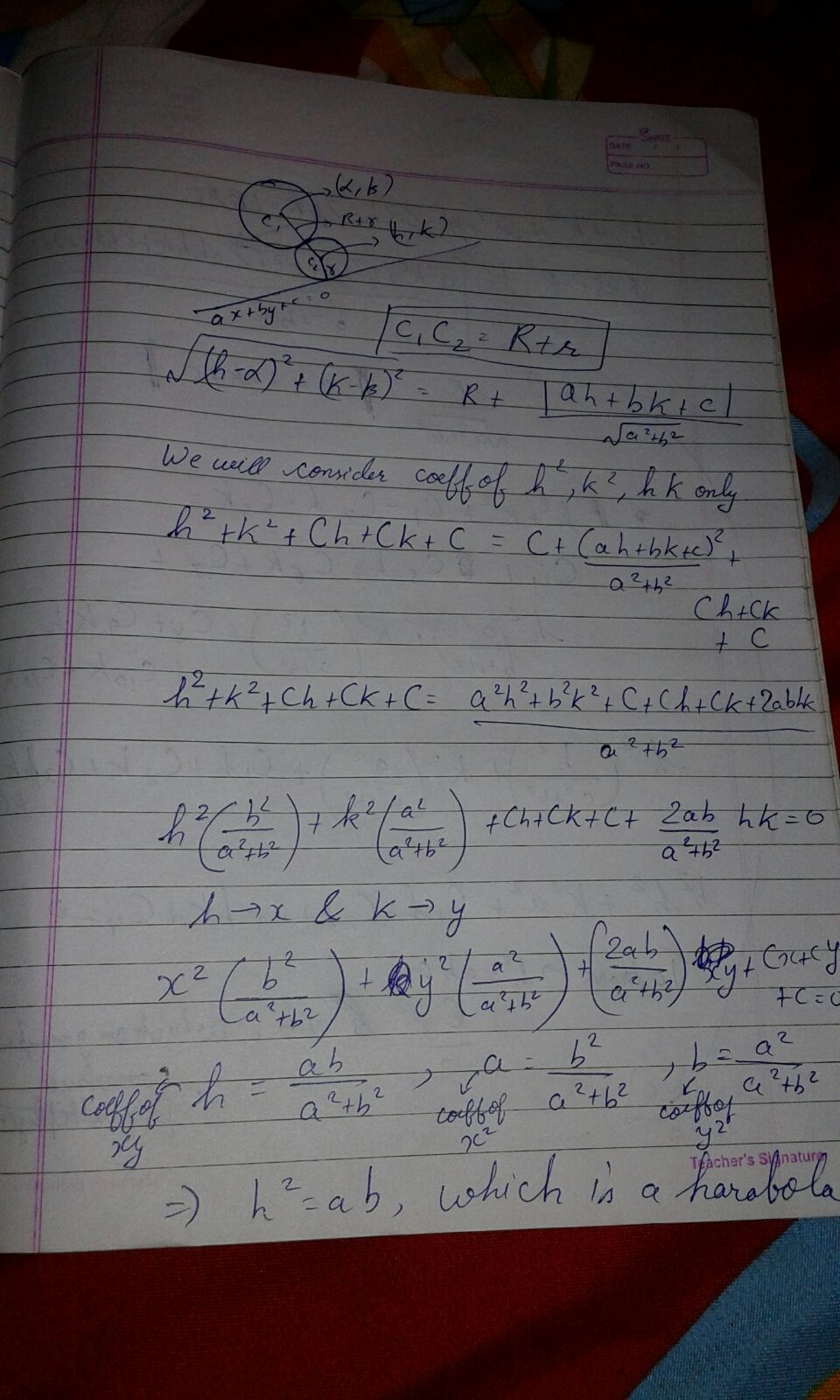- 4 years, 9 months ago

See the bedsheet behind the register, its also same! How can this be possible?

- 4 years, 9 months ago

Alternate universes.- 4 years, 9 months ago

Its mine solution. So thats cheating.

- 4 years, 9 months ago

You just copied his solution :P

- 4 years, 9 months ago

well,didn't you do!

- 4 years, 9 months ago

Post the next.

- 4 years, 9 months ago

i wrote it.How can you say that?our handwriting is same

- 4 years, 9 months ago

Well I took his solution and your solution and compared it pixel by pixel and compared colours. It is also mesmerizing that you took the photo at the same angle and from the smae distance and under the same light and in the same notebook of his. What is even more surprising? The solutions are the same.

_<

- 4 years, 9 months ago

One more thing see the bedsheet behind the register, Its also same

- 4 years, 9 months ago

Haha. Its mine. I can identify my writing

- 4 years, 9 months ago

Maybe you two are the same people from alternate universes. :P

- 4 years, 9 months ago

maybe

- 4 years, 9 months ago

So, do I get the points for it?

- 4 years, 9 months ago

no

- 4 years, 9 months ago

K, that's fine as long as problem remains.

- 4 years, 9 months ago

He is a bad person. See he awarded marks to himself

- 4 years, 9 months ago

Problem looked familiar. https://brilliant.org/problems/locus-of-circles-centre/

- 4 years, 9 months ago

You did a wrong thing by posting the link. You should have written your solution.

- 4 years, 9 months ago

Would've taken too long. Sorry. Also, I recognised the question (Eidetic/Photographic memory).

- 4 years, 9 months ago

Try the proof without seeing. Its great really

- 4 years, 9 months ago

But the proof is great.

- 4 years, 9 months ago

True, but I found this problem not too hard to show via co-ord geom. Still, my problem to post?

- 4 years, 9 months ago

Problem 7 [3 points] Suppose the medians of a triangle are 5,12,13 units.Find the sides of the triangle.

- 4 years, 9 months ago

26/3,2(sqrt244)/3,2(sqrt601)/3

- 4 years, 9 months ago

ya! sharky is right.@Prince Loomba post your solution.

- 4 years, 9 months ago

Its a direct formula. Side=$\frac {2}{3} \times \sqrt {2 ((m_{a})^2+(m_b)^{2})-{m_{c}}^2}$

- 4 years, 9 months ago

oh..thats nice

- 4 years, 9 months ago

Why am I awarded points even though I didn't posted the solution to previous problem?

- 4 years, 9 months ago

you didn't.really?

- 4 years, 9 months ago

BTW, my method is using formula $AB^2 + AC^2 = 2(AD^2 + BD^2)$, then using simultaneous linear equations.

- 4 years, 9 months ago

Even i used the same thing.

- 4 years, 9 months ago

Beat me to it!!! Can we please post proofs of why it is true?

- 4 years, 9 months ago

Post next bro.

- 4 years, 9 months ago

correct!

- 4 years, 9 months ago

Problem 6 [2 points]

What is the area of the largest circle possible with center at coordinate (0,0), but doesn't intersect the area bounded by $y>\frac{1}{\left|x\right|}$?

- 4 years, 9 months ago

Simple, easy question. We wish to find the shortest distance between the Origin and this graph. But this can be easily done as follows: We have $y = \frac{1}{|x|}$ and we wish to minimise $x^2 + y^2$ (Using distance formula). Substituting $y^2 = \frac {1}{x^2}$, we have $x^2 + \frac {1}{x^2}$. Note that $x^2$ is non-negative, so we can apply AM-GM to get that its minimum value is $2$. Thus, the minimum distance between the curve and the origin is $\sqrt{2}$. Thus, the largest circle satisfying has a radius of $\sqrt{2}$, so it has an area of $2\pi$.

- 4 years, 9 months ago

I have a short trick. The nearest point is (1,1) and (-1,1). And if circle is bigger than that, then it will be excluded. So circle covering 1,1 and center origin area is 2pi

- 4 years, 9 months ago

Is the ander 2pi?

- 4 years, 9 months ago

Post next bro

- 4 years, 9 months ago

Sorry my net was down a bit.

- 4 years, 9 months ago

Correct!

- 4 years, 9 months ago

Same here, 2pi

- 4 years, 9 months ago

Problem 5 ($x-1$ marks)

An equilateral triangle of side $1$ has a circle inscribed in it, so that it touches all sides of the triangle at their medians. An equilateral triangle is inscribed in the circle.

The process of circle inscribing and triangle inscribing is repeated until infinity. Find the exact value of the sum of the areas of all triangles.

If the value is in the form $\dfrac{\sqrt{x}}{y}$, find $x+y$.

- 4 years, 9 months ago

The answer is $\frac{\sqrt{3}}{3}$.

Each smaller triangle's area can be seen to be 1/4 of the triangle bigger than it.

For instance, the first triangle's area is $\sqrt{3}/4$. THe second triangle would be that area divided by 4. The third triangle would be the second divided by four again and so on.

Hence, summing all the areas, the total area gives $\frac{\sqrt{3}}{3}$

- 4 years, 9 months ago

@Julian Poon the answer is 6 not sqrt3÷3 you have to x+y not the whole. But that doesnt matter because your solution is right.

- 4 years, 9 months ago

Ah well...

- 4 years, 9 months ago

Correct! $x-1$ marks for you good sir.

- 4 years, 9 months ago

Haha x-1 marks is x used up in the question, right?

- 4 years, 9 months ago

Indeed $:)$

- 4 years, 9 months ago

Problem 4 [2 points]

Suppose your house is at coordinate (1,1)

You want to visit your friend's house which is located at coordinate (-3, 5)

However, you want to first walk towards the sea to collect seashells, and then walk to your friend's house.

The coastline is described as the line $y=0$, you can visit any point of the line to collect your seashells.

Now, what is the minimum distance you would have to walk?

- 4 years, 9 months ago

My point is if you were to only go to your friends house you d have to cross the y axis. then you the minimum distance should be the just the distance from your house to your house which can be solved by pythagoras theorem. if the distance was a. then i have

a^2 = 2×4^2. because the difference between x and y coordinates of both x and y is the same which is 4.

Solving we have a=sqrt 32

- 4 years, 9 months ago

Yes I also think this

- 4 years, 9 months ago

The x- axis is the coastline and not y- axis.

- 4 years, 9 months ago

Right. Its sqrt 52

- 4 years, 9 months ago

The answer is $\sqrt{52}$ or $2\sqrt{13}$

- 4 years, 9 months ago

Oh boy, that means I have to post another one

- 4 years, 9 months ago

$2\sqrt{13}$

Reflect the point $(-3,5)$ in the $x$ axis and walk from $(1,1)$ to $(-3,-5)$ in a straight line. Using Pythagoras this is $\sqrt{6^2+4^2}=\sqrt{52}=\large \color{#20A900}{\boxed{2\sqrt{13}}}$. Now reflect the part of the line below the $x$ axis in the $x$ axis, and this is the minimum distance

- 4 years, 9 months ago

Question 3 (3 marks)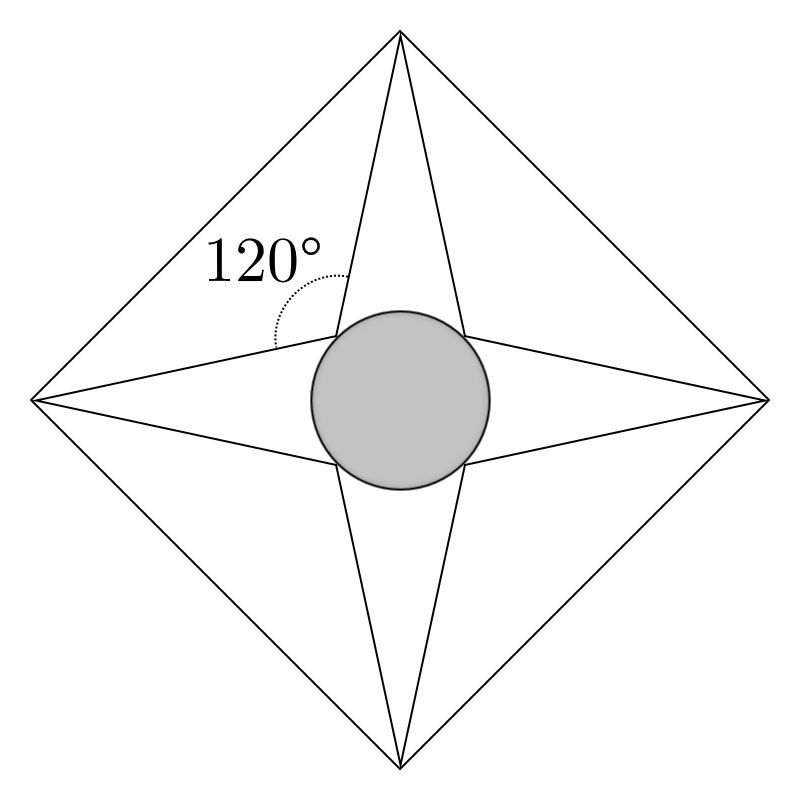The above figure shows a medal, comprised of a square of side $1$ with a four-pointed star inscribed in it, such that the angle between the points is $120^{\circ}$ as shown.

If the area of the circle inscribed in the star is $\dfrac{a-\sqrt3}{b} \pi$ for integers $a$ and $b$, find $ab$.

- 4 years, 9 months ago

Area is $\frac{2-\sqrt3}{6}\pi$ thus , answer is $\color{#20A900}{\boxed{12}}$

- 4 years, 9 months ago

Correct, but Julian answered first $:)$

- 4 years, 9 months ago

- 4 years, 9 months ago

To see why, the radius of the circle is half the length of the side minus the height of the triangle.

This gives

$r=\frac{1}{2}-\frac{1}{\sqrt{3}}$

THe area of the circle is $\pi r^2$

THis gives the area of the circle:

$\frac{2-\sqrt{3}}{6}*\pi$

- 4 years, 9 months ago

Post next

- 4 years, 9 months ago

Winner!

- 4 years, 9 months ago

Its 2D ?

And only of 3 marks?

- 4 years, 9 months ago

Yes, the figure is 2D. My solution is not too complicated, but also harder than your 2 marker, hence I will reward 3 points for it

- 4 years, 9 months ago

Haha TIT FOR TAT

I posted 2 marks and you 3 marks comparing with me

- 4 years, 9 months ago

Question 2 (2 marks)Find perimeter of the figure.

- 4 years, 9 months ago

The above figure is a square with an equilateral triangle $AED$ "indented" into it, since all angles are $60^{\circ}$. Therefore $AE=ED=AD=12$, and the perimeter is $5 \times 12 = \large \color{#20A900}{\boxed{60}}$.

- 4 years, 9 months ago

Nice solution. I was thinking of a solution when you posted this. You win haha :P

- 4 years, 9 months ago

Hehe. Posted the next problem.

- 4 years, 9 months ago

Right Post next problem

- 4 years, 9 months ago

Question 1[3marks]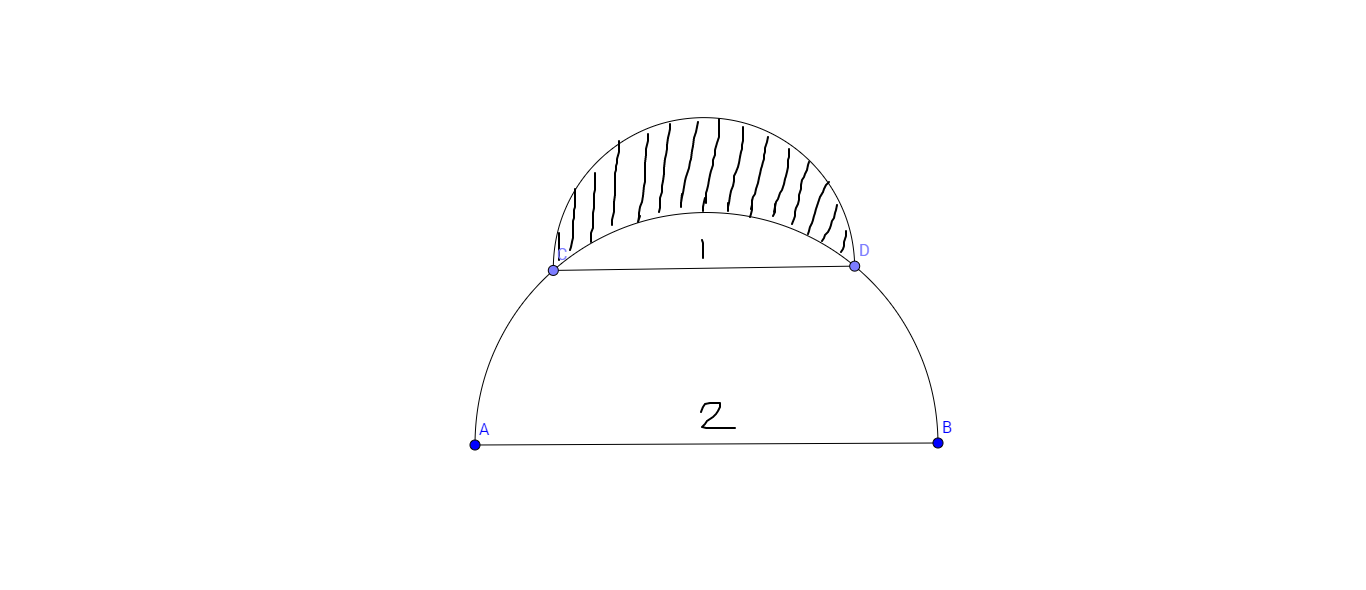A semicircle of diameter $1$ sits at the top of a semicircle of diameter $2$,as shown.The shaded area inside the smaller semicircle and outside the larger semicircle is called lune.Determine the area of the lune.

- 4 years, 9 months ago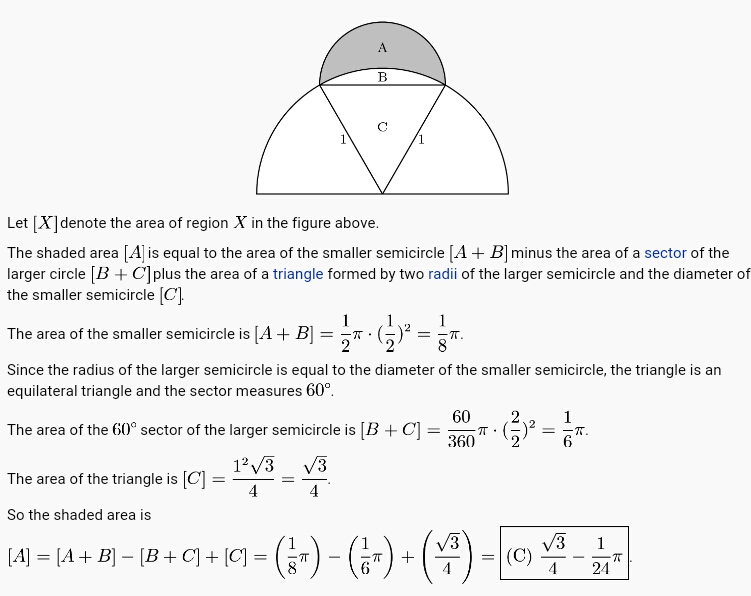- 4 years, 9 months ago

- 4 years, 9 months ago

Thanks, I will post new soon

- 4 years, 9 months ago